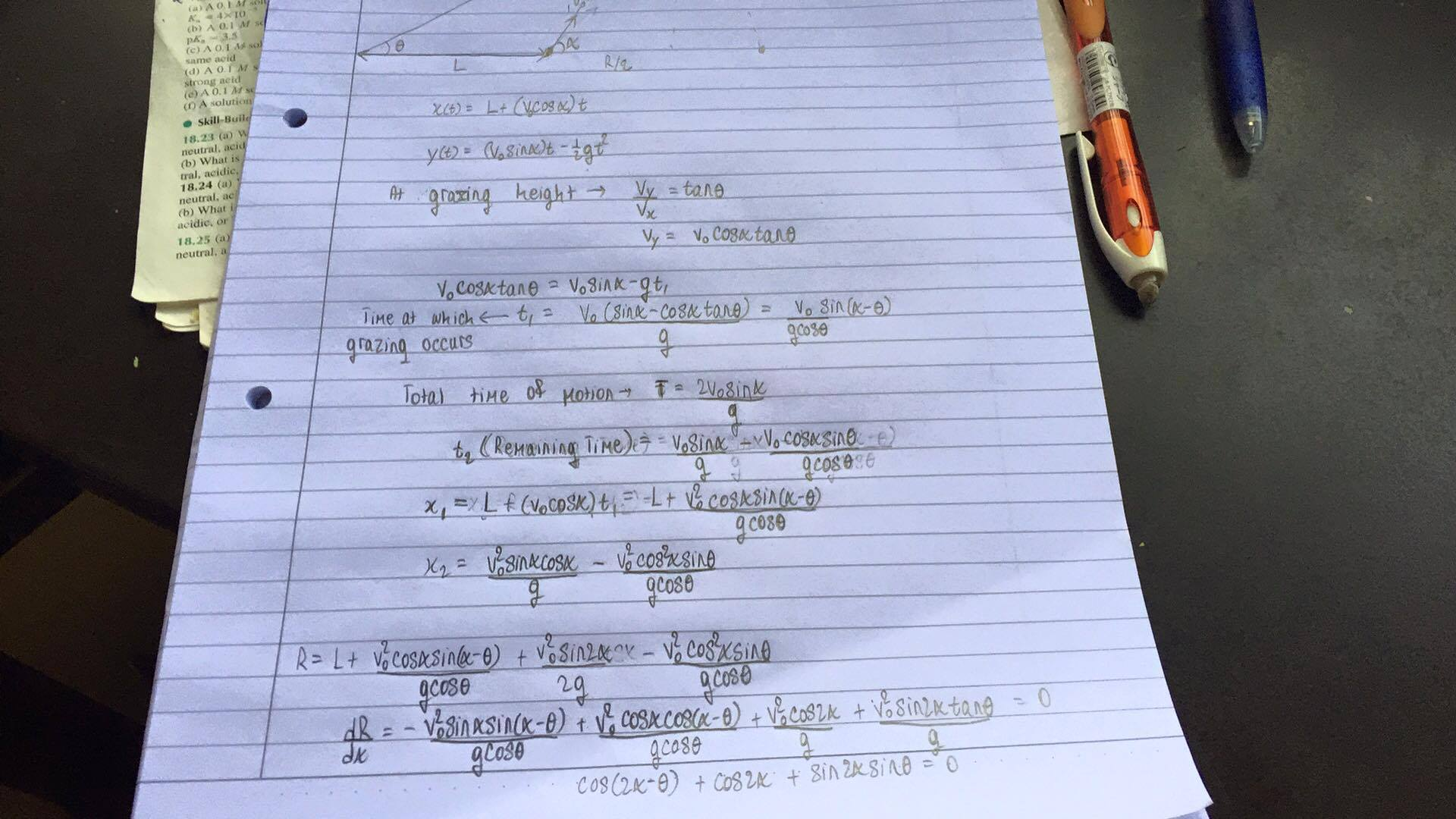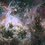# Problem in Projectile MotionSo, I've actually attached the diagram illustrating the problem and my attempt at it. The problem goes like this; I have a cave which is inclined at theta. I have a ball inside the cave which is at a distance $L$ from the innermost portion of the cave. I kick the ball at a velocity $v_0$ and at an angle alpha to the horizontal. Find $\alpha$, in terms of $L$, $v_0$ and $\theta$, such that the range is maximized.

Could someone help me out, please? Having a bit of difficulty visualizing what this would look like.Note by Abhijeet Vats
5 years, 1 month ago

This discussion board is a place to discuss our Daily Challenges and the math and science related to those challenges. Explanations are more than just a solution — they should explain the steps and thinking strategies that you used to obtain the solution. Comments should further the discussion of math and science.

When posting on Brilliant:

• Use the emojis to react to an explanation, whether you're congratulating a job well done , or just really confused .
• Ask specific questions about the challenge or the steps in somebody's explanation. Well-posed questions can add a lot to the discussion, but posting "I don't understand!" doesn't help anyone.
• Try to contribute something new to the discussion, whether it is an extension, generalization or other idea related to the challenge.
• Stay on topic — we're all here to learn more about math and science, not to hear about your favorite get-rich-quick scheme or current world events.

MarkdownAppears as
*italics* or _italics_ italics
**bold** or __bold__ bold
- bulleted- list
• bulleted
• list
1. numbered2. list
1. numbered
2. list
Note: you must add a full line of space before and after lists for them to show up correctly
paragraph 1paragraph 2

paragraph 1

paragraph 2

[example link](https://brilliant.org)example link
> This is a quote
This is a quote
    # I indented these lines
# 4 spaces, and now they show
# up as a code block.

print "hello world"
# I indented these lines
# 4 spaces, and now they show
# up as a code block.

print "hello world"
MathAppears as
Remember to wrap math in $$ ... $$ or $ ... $ to ensure proper formatting.
2 \times 3 $2 \times 3$
2^{34} $2^{34}$
a_{i-1} $a_{i-1}$
\frac{2}{3} $\frac{2}{3}$
\sqrt{2} $\sqrt{2}$
\sum_{i=1}^3 $\sum_{i=1}^3$
\sin \theta $\sin \theta$
\boxed{123} $\boxed{123}$

## Comments

Sort by:

Top Newest

Bump. Could someone help me out lol

- 5 years, 1 month ago

Log in to reply

Like in many mathematical problems there are multiple ways to approach it. First lets summarize the assumptions made to simplify this problem:

• The effect of air friction is negligible
• The free-fall acceleration is constant
• The ball is considered a singularity

Before diving into this particular problem, let's first have a look at the trajectory of the ball on itself.

In general the horizontal motion of a projectile is described by:

$x(t) = x_0 + \vec{v_0} \, cos(\alpha) \, t$

And its vertical motion by:

$y(t) = y_0 + \vec{v_0} \, \sin(\alpha) \, t - \frac{1}{2} \, g \, t^2$

where $\vec{v_0}$ (or just $v$ for convenience) is the initial velocity vector at an angle $\alpha$ to the horizontal. We can neglect $y_0$ because the ball is launched from the floor of the cave.

Notice you denoted $x_0$ as $L$ as being the horizontal distance from the innermost point of the cave to the point at which the ball was launched.

These expressions could be rewritten into a single expression describing the height $y$ in terms horizontal distance $x$ resulting in:

$y(x) = (x - L) \, \frac{v \, sin(\alpha)}{v \, cos(\alpha)} - \frac{g \, (x-L)^2}{2 \, (v \, cos(\alpha))^2}$

Simplified:

$y(x) = (x - L) \, tan(\alpha) - \frac{g \, (x-L)^2}{2 \, (v \, cos(\alpha))^2}$

The next step is to investigate the conditions at which the range is maximized. Therefore we need to look at the first two expressions again. When the ball hits the ground after its flight the height is equal to zero resulting in the following equation:

$y(t) = v \, \sin(\alpha) \, t - \frac{1}{2} \, g \, t^2 = 0$

This gives us two solutions:

$t = 0$

or

$t = \frac{2 \, v \, sin(\theta)}{g}$

It can be easily seen that we were looking for the latter solution. Substituting this solution in the very first equation gives:

$x - L = \frac{2 \, v^2 \, \cos(\alpha) \, \sin(\alpha)}{g}$

Using the trigonometric identity:

$sin(2\alpha) = 2 \, sin(\alpha) \, cos(\alpha)$

simplifies this to:

$x - L = \frac{v^2 \, sin(2\alpha)}{g}$

Notice that $x - L$ is the horizontal distance traveled by the ball and it's maximized when the angle $\alpha$ equals $45^{\circ}$.

Now we can dive into this particular problem. One way to approach this problem is by calculating the point at which the slope of the trajectory of the ball is the same as the slope of cave's ceiling. With this knowledge it's possible to calculate the horizontal shifting $L$ of the trajectory needed to let the ball not hit the cave's ceiling. The height of the cave's ceiling at distance $x$:

$y_c(x) = x \, tan(\theta)$

And its slope:

$\frac{d y_c}{d x} = tan(\theta)$

The height of the ball at distance $x$ as found earlier:

$y_b(x) = x \, tan(\alpha) - \frac{g \, x^2}{2 \, (v \, cos(\alpha))^2}$

Notice $L = 0$ since the horizontal shifting is calculated later on.

And its slope:

$\frac{d y_b}{d x} = tan(\alpha) - \frac{g \, x}{(v \, cos(\alpha))^2}$

Equal slopes at:

$tan(\alpha) - \frac{g \,x}{(v \, cos(\alpha))^2} = tan(\theta)$

Solving for $x$:

$s = x = \frac{v^2 \, cos(\alpha) \, sin(\alpha - \theta)}{g \, cos(\theta)} \quad and \quad g \neq 0 \quad and \quad v \neq 0$

Now it's known at what horizontal distance the slope of the trajectory will be equal to the slope of the cave's ceiling. The difference in height between the trajectory of the ball and the cave's ceiling can be converted into a horizontal distance that the trajectory needs to shift in order to let the ball not hit the ceiling. The horizontal shifting in terms of difference in height can be derived from the slope of the cave's ceiling:

$L = \frac{|y_c(s) - y_b(s)|}{tan(\theta)}$

This simplifies to:

$L = \frac{v^2 \, sin^2(\alpha-\theta))}{g \, sin(2 \, \theta)}$

Although it is not in explicit for $\alpha$, I think this equation is quite elegant.

Here's a plot of a fitted trajectory with $v = 10 \, ms^{-1}, \alpha = 45^{\circ}, \theta = 15^{\circ}$ and $g = 9.81 \, ms^{-2}$:Fitted Trajectory

And ofcourse to check:

$L = \frac{10^2 \cdot sin^2(45-15)}{9.81 \cdot sin(2 \cdot 15)} = 5.097$

For a maximized range $\alpha$ has to be as close as possible to $45^{\circ}$. Notice that $\alpha$ would never be larger than $45^{\circ}$ for a maximized range.

- 5 years, 1 month ago

Log in to reply

Thank you so much for your solution! Could you explain the last part of the solution though? Like, the part about the horizontal shifting. I didn't quite understand them properly.

- 5 years, 1 month ago

Log in to reply

Imagine yourself that the ball was kicked from the innermost position of the cave ( $L=0$ ) . When the ball was kicked under an angle $\alpha$ larger than $\theta$, the ball would bounce against the ceiling (red line in plot) . Since we don't want this to happen for a maximized range we shift the initial position where the ball was kicked so the ball wouldn't hit the ceiling (green line in plot).

- 5 years, 1 month ago

Log in to reply

×

Problem Loading...

Note Loading...

Set Loading...